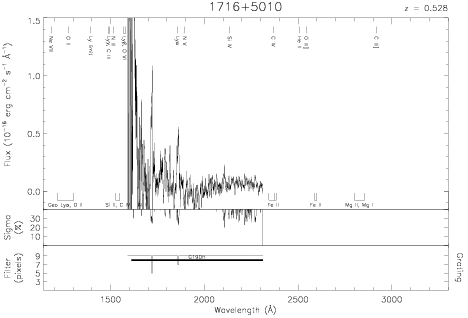Download dataset
TELESCOP= 'HST     '
INSTRUME= 'FOS     '
EQUINOX =               2000.0
DATE-OBS= '1992-09-28T04:29:03'
RA_PNT  =       259.4721980518
DEC_PNT =        50.1310104355
PA_PNT  =       174.7367000000
OBJECT  = '1716+5010'
ROOTNM01= 'Y1460902T'
GRNDMD01= 'SPECTROSCOPY'
DETECT01= 'AMBER   '
APERID01= 'A-1     '
FGWAID01= 'H19     '
ROOTNM02= 'Y1460903T'
GRNDMD02= 'SPECTROSCOPY'
DETECT02= 'AMBER   '
APERID02= 'A-1     '
FGWAID02= 'H19     '
ROOTNM03= 'Y1460904T'
GRNDMD03= 'SPECTROSCOPY'
DETECT03= 'AMBER   '
APERID03= 'A-1     '
FGWAID03= 'H19     '
ROOTNM04= 'Y1460905T'
GRNDMD04= 'SPECTROSCOPY'
DETECT04= 'AMBER   '
APERID04= 'A-1     '
FGWAID04= 'H19     '
ROOTNM05= 'Y1460A02T'
GRNDMD05= 'SPECTROSCOPY'
DETECT05= 'AMBER   '
APERID05= 'A-1     '
FGWAID05= 'H19     '
ROOTNM06= 'Y1460A03T'
GRNDMD06= 'SPECTROSCOPY'
DETECT06= 'AMBER   '
APERID06= 'A-1     '
FGWAID06= 'H19     '
ROOTNM07= 'Y1460A04T'
GRNDMD07= 'SPECTROSCOPY'
DETECT07= 'AMBER   '
APERID07= 'A-1     '
FGWAID07= 'H19     '
ROOTNM08= 'Y1460A05T'
GRNDMD08= 'SPECTROSCOPY'
DETECT08= 'AMBER   '
APERID08= 'A-1     '
FGWAID08= 'H19     '